# Introduction

Most spatial modeling approaches work under the assumption that the data used for modeling are stationary. That is to say, the mean and variance of the response variable are constant in space. This assumption is often violated, especially when modeling large areas. Sometimes, a nonstationary process can be transformed into a stationary one by modeling external trends; however, this is not possible to achieve if the external trends are not constant over space. In such cases, an alternative approach is to partition the space into stationary sub-regions and model each region separately. The problem with this approach is that continuous response variables will have discontinuities in the prediction surface at the borders of the regions.

The package remap is an implementation of a regional modeling with border smoothing method that results in a continuous global model. Border smoothing is accomplished by taking a weighted average of regional model predictions near region borders. The remap function is also a convenient way to build independent models in a partitioned space, even if no border smoothing is required for the problem.

library(magrittr) # For pipe %>% functionality
library(tibble)   # For light data wrangling
library(dplyr)    # For light data wrangling
library(ggplot2)  # For plots
library(maps)     # For a polygon of the state of Utah
library(sf)       # For spatial data manipulation
library(mgcv)     # For GAM modeling

library(remap)
data(utsnow)
data(utws)

# Reset CRS in case user has old version of GDAL
sf::st_crs(utsnow) <- 4326
sf::st_crs(utws) <- 4326

## Data

To introduce the functionality of remap, we will look at a modeling problem for estimating snow water content in the state of Utah using water equivalent of snow density (WESD) measurements. The utsnow data that is part of the package remap contains WESD in mm water measured on April 1st, 2011 at 394 locations within and near the state of Utah. The utws data in remap is a set of polygons representing watersheds defined by the US Geological Survey. These watersheds are defined by a hierarchy of hydrologic unit cods (HUC) with a two-digit designation for continental scale watersheds (HUC2). We will build a regionalized model with remap using HUC2 watershed regions.

utstate <- maps::map("state", plot = FALSE, fill = TRUE) %>%
sf::st_as_sf() %>%
dplyr::filter(ID == "utah") %>%
sf::st_transform(crs = 4326)

ggplot(utws, aes(fill = HUC2)) +
geom_sf(alpha = 0.5) +
geom_sf(data = utstate, fill = "NA", size = 1) +
geom_sf(data = utsnow) +
ggtitle("Modeling Data and Regions",
"HUC2 regions are made up of smaller HUC4 regions.") +
theme_void()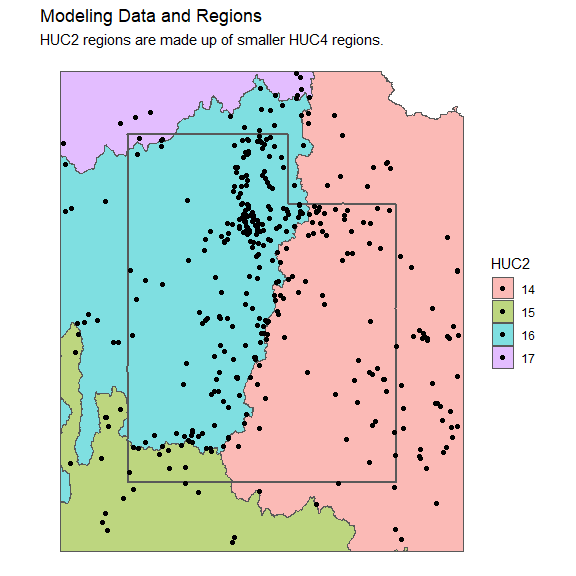$$~$$

$$~$$

$$~$$

# Basic Models

The remap function requires the following 4 parameters:

• data - Observations with a spatial component used for modeling.
• regions - Polygons describing the regions used to build each model.
• model_function - A function that takes a subset of the data and returns a model.
• buffer - Observations are located within and near a region are used to build each model. The buffer parameter dictates how near an observation must be to a location to be included in that region’s model.

The following parameters are optional:

• region_id - A column name of regions which describes which polygons to include in each region. This is helpful if any region has polygons described in multiple rows in the sf object. The region_id allows for combinations of smaller polygons into larger regions.
• min_n - The minimum number of observations required to build a model in each region. If a region does not contain enough observations within its border and buffer zone, the nearest min_n observations will be used for modeling.

In this section, we use some simple linear models to model snow water content in Utah. First a global model using all data, then a regional model using remap. The WESD measurement in our example data commonly shares a log-linear relationship with elevation in mountainous western states. Many locations in Utah have a value of 0 for WESD on April first. Since zero snow values in log transformed variables add another level of complexity to the modeling process, we remove them for now and make a new dataset called utsnz.

utsnz <- utsnow %>% dplyr::filter(WESD > 0)

## Global Linear Model

The relationship between the log transformed WESD and elevation of utsnz is visualized as:

ggplot(utsnz, aes(x = ELEVATION, y = WESD)) +
geom_point() +
geom_smooth(method = "lm") +
scale_y_log10() +
theme_minimal()
#> geom_smooth() using formula 'y ~ x'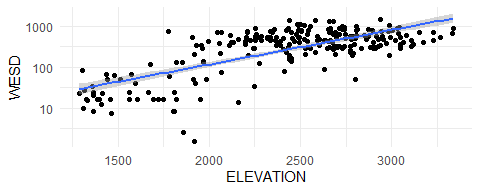The resubstitution mean squared error (MSE) for such a model is:

lm_global <- lm(log(WESD) ~ ELEVATION, data = utsnz)

lm_global_mse <- mean((utsnz$WESD - exp(predict(lm_global, utsnz)))^2) lm_global_mse #>  121370.7 ## Regionalized Linear Models The utsnz data describes which watersheds each location falls in with the HUC2 columns. (Note that remap does not require these columns in the data when building models using the utws regions.) Here is what the relationship between log(WESD) and elevation looks like for each of the HUC2 regions: ggplot(utsnz, aes(x = ELEVATION, y = WESD)) + facet_grid(HUC2 ~ .) + geom_point() + geom_smooth(method = "lm") + scale_y_log10() + theme_minimal() #> geom_smooth() using formula 'y ~ x'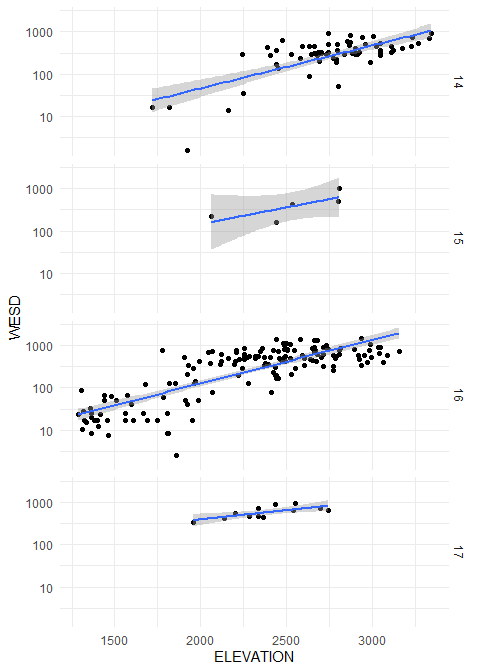The linear models for each HUC2 region seem to fit a little better than the global linear model; however, it looks like HUC2 region 15 does not have enough data to build a very confident model. Using remap to build models for each region, some of the nearest observations to HUC2 region 15 are added to build a better model by using the min_n parameter to set the minimum number of observations per region to 10. For this modeling task, any observation within 20km of a region is to be included in that region’s model using the buffer parameter. The lm function requires a formula, so formula is added as an extra parameter in remap. Since remap makes smooth predictions over the entire surface, a smooth parameter is required in the predict function that dictates the distance from region borders where the smoothing process will start. We set smooth to 10km for this example. t1 <- Sys.time() lm_huc2 <- remap::remap( utsnz, utws, region_id = HUC2, buffer = 20, min_n = 10, model_function = lm, formula = log(WESD) ~ ELEVATION ) lm_huc2_mse <- mean((utsnz$WESD - exp(predict(lm_huc2, utsnz, smooth = 10)))^2)
t2 <- Sys.time()

# mse
lm_huc2_mse
#>  85725.36

# runtime
round(difftime(t2, t1), 1)
#> Time difference of 22.9 secs

The output of remap returns a list that contains a list of models built in each region and an sf data frame storing the region polygons. The models for each region can be accessed directly. For example, the coefficients for each model can be accessed with the following code:

sapply(lm_huc2$models, function(x) x$coefficients)
#>                      14           15          16        17
#> (Intercept) 0.557864695 -2.292085274 0.564719359 1.8300546
#> ELEVATION   0.001914744  0.003114987 0.002140786 0.0018686

The resulting remap model has a 29.4% reduction in resubstitution MSE using separate linear models for each of the HUC2 regions rather than the global linear model. This increase in accuracy is likely to be even more drastic when problems span greater areas, such as a model for an entire continent.

$$~$$

$$~$$

$$~$$

# Computational Speedup

Many modeling techniques that partition space depend on the distance between prediction locations and the center of each region. However, the center is a poor characterization of irregularly shaped regions. The border smoothing method in remap uses the distances to region borders rather than region centers. This allows for regions of any shape being used for modeling. Building models and predicting with remap runs fairly slowly for something as simple as linear models. This is because remap requires distance calculations between each observation and the region boundaries in both the modeling and prediction steps. This tends to be computationally expensive with large numbers of points and/or complex region boundaries. This section outlines the steps taken to ameliorate the computational burden of remap which allow remap to scale to large problems.

## Simplifying Regions

Many spatial processes require continuous polygons (i.e. no gaps in region boundaries), which limits the degree of polygon simplification that can be achieved. One desirable feature of remap is the ability of each model to make smooth predict outside of polygons within a smoothing zone, which smooths over any small gaps in polygons that occurs in an aggressive geometrical simplification. Thus, by only preserving the macro structure of the input polygons, we can greatly speed up distance computations without losing fidelity in model predictions.

Distance calculation time can be dramatically reduced by simplifying the polygons passed to remap using the sf package st_simplify function. The function gives a warning that can be ignored for our purposes, we are only trying to preserve the macro structure and the regions are not near any poles. Gaps at region borders appear, but remap has the ability to predict outside of regions and predictions will remain smooth as long as the gaps aren’t wider than two times the smooth parameter. Notice how the simplified polygons retain the basic structure of the original regions:

utws_simp <- utws %>% sf::st_simplify(dTolerance = 0.05)
#> Warning in st_simplify.sfc(st_geometry(x), preserveTopology, dTolerance):
#> st_simplify does not correctly simplify longitude/latitude data, dTolerance
#> needs to be in decimal degrees

rbind(
utws %>% dplyr::mutate(TYPE = "Original Watershed Polygons"),
utws_simp %>% dplyr::mutate(TYPE = "Simplified Watershed Polygons")
) %>%
ggplot() +
facet_grid(.~TYPE) +
geom_sf() +
theme_void()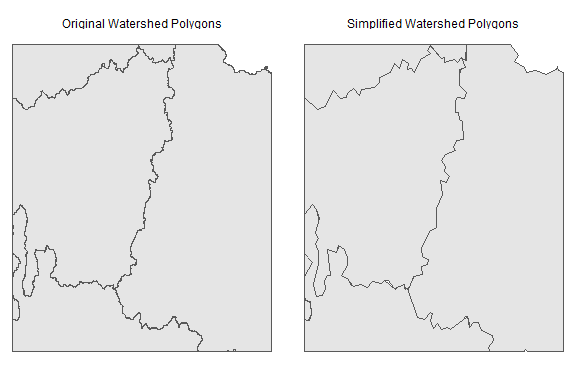Simplifying the polygons doesn’t drastically change the computation time in this particular example, but some regions can contain massive polygons with details that are unnecessary for regional modeling.

## redist

The remap and predict functions both internally call a function called redist to calculate distances from points to polygons. The user can directly use the function redist to pre-compute distances from points to polygons and use the pre-computed distances as direct inputs in the remap function. The pre-computing step greatly reduces computational costs if multiple regional models must be made with the same input data and polygons.

Distances from prediction locations need only be computed to polygon boundaries for which the prediction location falls within their smoothing zone. Buffered polygons can be used to quickly determine candidate observations for distance calculations in each region. The max_dist parameter of redist can be used to make these buffered polygons. This drastically reduces the number of points for which distance calculations must be performed and greatly improves computation times.

huc2_dist_nz <- remap::redist(utsnz, utws_simp, region_id = HUC2)
head(huc2_dist_nz)
#> Units: [km]
#>            14       15       16        17
#> [1,]  0.00000 330.5847 272.5228 437.81560
#> [2,]  0.00000 428.2982 247.8652 358.01516
#> [3,]  0.00000 236.7842 285.3623 526.89371
#> [4,] 74.03409 386.9778   0.0000  64.61556
#> [5,] 76.54282 324.1539   0.0000 152.82797
#> [6,] 97.67083 175.9193   0.0000 284.43786

The newly created distance matrix can be sent to remap and predict as a parameter. Run the following code and notice how much faster the remap and predict functions run when the distances are pre-calculated.

t1 <- Sys.time()
lm_huc2 <- remap(
utsnz, utws_simp, region_id = HUC2,
buffer = 20, min_n = 10,
model_function = lm,
formula = log(WESD) ~ ELEVATION,
distances = huc2_dist_nz
)

lm_huc2_mse <- mean(
(utsnz$WESD - exp(predict(lm_huc2, utsnz, smooth = 10, distances = huc2_dist_nz)))^2 ) t2 <- Sys.time() # mse lm_huc2_mse #>  86925.69 # runtime round(difftime(t2, t1), 1) #> Time difference of 0.1 secs The MSE for this model is slightly different than previous regional linear model since the simplified polygons are being used. ## Parallel Code Since calculating distances to polygons is independent for each polygon, redist can be run in parallel by setting the cores to a number greater than one. Models are built and make predictions independent of one another so the remap function and predict method also have a cores parameter for parallel computing. This means that distance calculations, modeling, and predicting processes can all be run in parallel. $$~$$ $$~$$ $$~$$ # Custom Models While linear models are great for illustration, many spatial modeling approaches will require more complex regional model forms. The remap function is flexible enough to handle arbitrary model inputs and outputs, with the only requirement being that the model output must be compatible for use with the generic predict function and return a numeric output. The remap function takes a model_function as a parameter. The model_function must have the following two features: • Its first unnamed function argument must take a subset of an sf data point object, • The function must output an object with a generic predict function that returns a vector of numeric values. Note that named function arguments can be supplied at the end of the remap function, as was the case with the formula argument in the linear model example shown previously. The key is that the first unnamed parameter be dedicated to data input. Suppose we want to make a generalized additive model (GAM) that is limited to only making positive predictions. We also don’t want to predict a number greater than the highest observed response in each region to avoid over extrapolation. This can be accomplished through wrapper functions for both gam and predict.gam that restrict predicted values to a specified range. gam_limit <- function(data, fml) { g_model <- mgcv::gam(fml, data = data) upper_lim <- max(data$WESD)

out <- list(g_model = g_model, upper_lim = upper_lim)
class(out) <- "gam_limit"
return(out)
}

predict.gam_limit <- function(object, newobs) {
if (nrow(newobs) != 0) {
preds <- predict(object$g_model, newobs) preds[preds < 0] <- 0 preds[preds > object$upper_lim] <- object$upper_lim return(preds) } return(NULL) } ## Global GAM Model The following code tests a GAM model where elevation and splines on the sphere are used as predictors. We can use the functions written to do a GAM with remap to easily do cross validation on a global model: # Create vector for cross-validation set.seed(42) cv_k <- sample(1:10, nrow(utsnow), replace = TRUE) # Initialize predictions gam_global_preds <- rep(as.numeric(NA), nrow(utsnow)) # Formula for global GAM global_fml <- WESD ~ s(ELEVATION, k = 5) + s(LATITUDE, LONGITUDE, bs = "sos", k = 50) # Build and test models with 10 fold cross-validation for (i in 1:10) { index <- cv_k == i gam_global <- gam_limit(utsnow[!index, ], fml = global_fml) gam_global_preds[index] <- predict(gam_global, utsnow[index, ]) } # Calculate MSE gam_global_mse <- mean((utsnow$WESD - gam_global_preds)^2)
gam_global_mse
#>  23875.94

This model is much better than either of the basic linear models, even though the GAM accuracy is measured on cross-validation rather than resubstitution error and the GAM model is also modeling the zero valued observations.

## Regionalized GAM Model

First, the distances are pre-calculated so the distance calculations aren’t repeated 10 different times when doing cross validation. The distances return a matrix where each row corresponds to each observation in the data. The cross validation only uses a subset of the data, so the corresponding subset of distances should be passed to remap during each modeling step.

huc2_dist <- remap::redist(utsnow, utws, region_id = HUC2)

HUC2 regions are used to build a regionalized GAM models with remap. We will reduce the knots on the splines on the sphere from 50 to 25 so we don’t need so many degrees of freedom for each model. The min_n can be set to 35 to allow at least 5 degrees of freedom per model.

# Initialize predictions
gam_huc2_preds <- rep(as.numeric(NA), nrow(utsnow))

# Formula for regional GAMs
gam_huc2_fml <- WESD ~ s(ELEVATION, k = 5) + s(LATITUDE, LONGITUDE, bs = "sos", k = 25)

# Build and test models with 10 fold cross-validation
for (i in 1:10) {
index <- cv_k == i

gam_huc2 <- remap::remap(
utsnow[!index, ], utws, region_id = HUC2,
model_function = gam_limit,
buffer = 20, min_n = 35,
distances = huc2_dist[!index, ],
fml = gam_huc2_fml
)

gam_huc2_preds[index] <- predict(
gam_huc2, utsnow[index, ],
smooth = 10,
distances = huc2_dist[index, ]
)
}

# Calculate MSE
gam_huc2_mse <- mean((utsnow\$WESD - gam_huc2_preds)^2)
gam_huc2_mse
#>  16620.54

The HUC2 regionalized GAM has 30.4% better MSE than the global GAM model.

$$~$$

$$~$$

$$~$$

# Smooth Predictions

A toy model is best used to show how smooth predictions work since the Utah snow water content models have extreme values and sharp changes with elevation. The toy model has 3 regional models with contrived response variables that consists of an affine combination of longitude and latitude values. The left region predicts $$lat - lon$$, the bottom region predicts $$lon - lat - 0.4$$, and the right region predicts $$lat - lon + 0.3$$

# Make regions
toy_regions <- tibble::tribble(
~id, ~geometry,
"a", sf::st_polygon(list(matrix(c(0, 0, 2, 0, 6, 3, 4, 10, 0, 10, 0, 0)*.1, ncol = 2, byrow = TRUE))),
"b", sf::st_polygon(list(matrix(c(2, 0, 10, 0, 10, 4, 6, 3, 2, 0)*.1, ncol = 2, byrow = TRUE))),
"c", sf::st_polygon(list(matrix(c(4, 10, 6, 3, 10, 4, 10, 10, 4, 10)*.1, ncol = 2, byrow = TRUE)))
) %>%
sf::st_as_sf(crs = 4326)

# Manually make a toy remap model
make_toy <- function(x) {
class(x) <- "toy_model"
return(x)
}
remap_toy_model <- list(
models = list("a" = make_toy("a"),
"b" = make_toy("b"),
"c" = make_toy("c")),
regions = toy_regions,
region_id = "id"
)
class(remap_toy_model) <- "remap"

# Make a prediction method for toy_model
predict.toy_model <- function(object, data) {
x <- sf::st_coordinates(data)[, "X"]
y <- sf::st_coordinates(data)[, "Y"]
if (object == "a") {
y - x
} else if (object == "b") {
x - y - 0.4
} else {
y - x + 0.3
}
}

# Make a grid over the regions for predictions
grd <- sf::st_make_grid(toy_regions, cellsize = .01, what = "corners") %>%
sf::st_sf()

The regions cover the following area:

ggplot2::ggplot(toy_regions, aes(fill = id)) +
geom_sf(color = "black", size = 1) +
ggtitle("Toy Regions") +
theme_bw()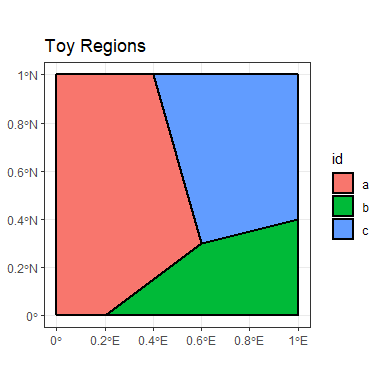The remap_toy_model object can now be used to make predictions on the grd object. There are 10201 points in the grd object but the regions are simple, so it will not take long to find distances. Two predictions will be made, the SHARP predictions will have a smoothing parameter of zero and the SMOOTH predictions will have a smoothing parameter set to 30km.

grd_pred <- grd %>%
dplyr::mutate(SHARP = predict(remap_toy_model, grd, smooth = 0),
SMOOTH = predict(remap_toy_model, grd, smooth = 30),
LON = sf::st_coordinates(.)[, "X"],
LAT = sf::st_coordinates(.)[, "Y"])

The smooth predictions from the remap object start to become a weighted average of regional predictions when the predictions are within 30km of a region border. The following plots show what is happening with both the SHARP and SMOOTH predictions at predicted values at all locations and specific plots along the 0.8 degree N line. Notice how the predictions at the borders of the toy regions are smoothed:

ggplot(toy_regions) +
geom_sf() +
geom_tile(data = grd_pred, aes(x = LON, y = LAT, fill = SHARP)) +
scale_fill_viridis_c(limits = c(-0.3, 1)) +
geom_hline(yintercept = 0.8) +
ggtitle("Sharp Predictions", "Black line corresponds to x-axis of the next plot.") +
xlab("") + ylab("") +
theme_bw()
#> Warning: range backtransformation not implemented in this coord; results may be
#> wrong.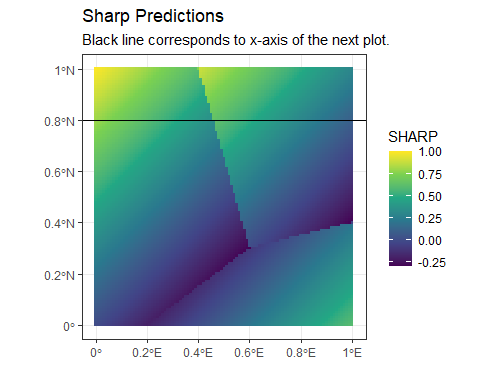ggplot(grd_pred %>% dplyr::filter(LAT == 0.8),
aes(x = LON, y = SHARP)) +
geom_line(size = 1) +
ggtitle("Sharp Predictions at 0.8 degrees N") +
theme_minimal()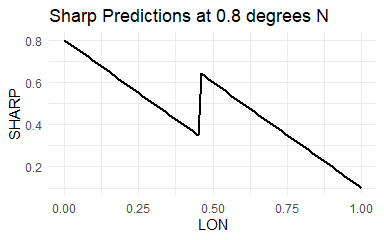ggplot(toy_regions) +
geom_sf() +
geom_tile(data = grd_pred, aes(x = LON, y = LAT, fill = SMOOTH)) +
scale_fill_viridis_c(limits = c(-0.3, 1)) +
geom_hline(yintercept = 0.8) +
ggtitle("Smooth Predictions", "Black line corresponds to x-axis of the next plot.") +
xlab("") + ylab("") +
theme_bw()
#> Warning: range backtransformation not implemented in this coord; results may be
#> wrong.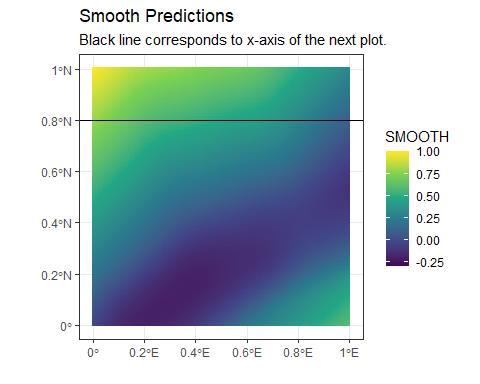ggplot(grd_pred %>% dplyr::filter(LAT == 0.8),
aes(x = LON, y = SMOOTH)) +
geom_line(size = 1) +
ggtitle("Smooth Predictions at 0.8 degrees N") +
theme_minimal()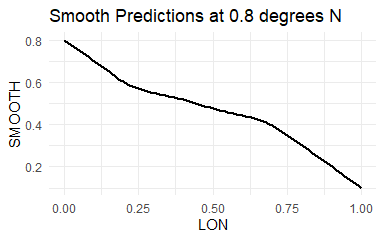The remap package provides a way to build regional spatial models given a set of observations and a set of regions. The resulting model can make predictions that have no discontinuities at region borders and scales well to large problems.